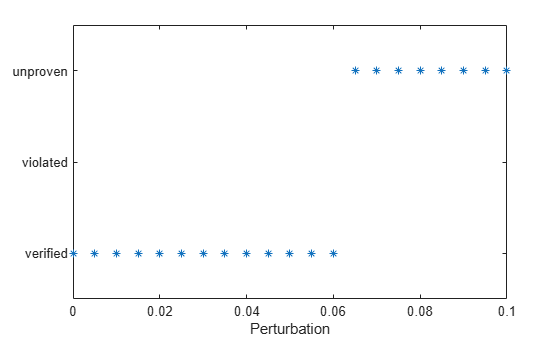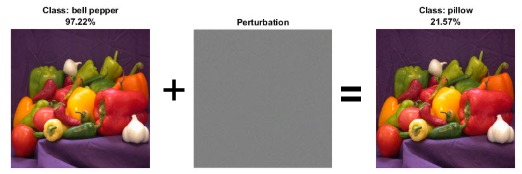# verifyNetworkRobustness

Verify adversarial robustness of deep learning network

Since R2022b

## Syntax

``result = verifyNetworkRobustness(net,XLower,XUpper,label)``

## Description

example

````result = verifyNetworkRobustness(net,XLower,XUpper,label)` verifies whether the network `net` is adversarially robust with respect to the class `label` when the input is between `XLower` and `XUpper`. For more information, see Adversarial Examples.A network is robust to adversarial examples for a specific input if the predicted class does not change when the input is perturbed between `XLower` and `XUpper`. For more information, see Algorithms.The `verifyNetworkRobustness` function requires the Deep Learning Toolbox Verification Library support package. If this support package is not installed, use the Add-On Explorer. To open the Add-On Explorer, go to the MATLAB® Toolstrip and click Add-Ons > Get Add-Ons.```

## Examples

collapse all

Verify the adversarial robustness of an image classification network.

Load a pretrained classification network. This network is a `dlnetwork` object that has been trained to predict the class label of images of handwritten digits.

`load("digitsRobustClassificationConvolutionNet.mat")`

Prepare the network for verification by removing the softmax layer. When you remove layers from a `dlnetwork` object, the software returns the network as an uninitialized `dlnetwork` object. To initialize the network, use the `initialize` function.

```netRobust = removeLayers(netRobust,"softmax"); netRobust = initialize(netRobust);```

`[XTest,TTest] = digitTest4DArrayData;`

Select the first ten images.

```X = XTest(:,:,:,1:10); label = TTest(1:10);```

Convert the test data to a `dlarray` object.

`X = dlarray(X,"SSCB");`

Verify the network robustness to an input perturbation between –0.01 and 0.01 for each pixel. Create lower and upper bounds for the input.

```perturbation = 0.01; XLower = X - perturbation; XUpper = X + perturbation;```

Verify the network robustness for each test image.

```result = verifyNetworkRobustness(netRobust,XLower,XUpper,label); summary(result)```
``` verified 10 violated 0 unproven 0 ```

Find the maximum adversarial perturbation that you can apply to an input without changing the predicted class.

Load a pretrained classification network. This network is a `dlnetwork` object that has been trained to predict the class of images of handwritten digits.

`load("digitsRobustClassificationConvolutionNet.mat")`

Prepare the network for verification by removing the softmax layer. When you remove layers from a `dlnetwork` object, the software returns the network as an uninitialized `dlnetwork` object. To initialize the network, use the `initialize` function.

```netRobust = removeLayers(netRobust,"softmax"); netRobust = initialize(netRobust);```

`[XTest,TTest] = digitTest4DArrayData;`

Select a test image.

```idx = 3; X = XTest(:,:,:,idx); label = TTest(idx);```

Create lower and upper bounds for a range of perturbation values.

```perturbationRange = 0:0.005:0.1; for i = 1:numel(perturbationRange) XLower(:,:,:,i) = X - perturbationRange(i); XUpper(:,:,:,i) = X + perturbationRange(i); end```

Repeat the class label for each set of bounds.

`label = repmat(label,numel(perturbationRange),1);`

Convert the bounds to `dlarray` objects.

```XLower = dlarray(XLower,"SSCB"); XUpper = dlarray(XUpper,"SSCB");```

Verify the adversarial robustness for each perturbation.

```result = verifyNetworkRobustness(netRobust,XLower,XUpper,label); plot(perturbationRange,result,"*") xlabel("Perturbation")```Find the maximum perturbation value for which the function returns `verified`.

```maxIdx = find(result=="verified",1,"last"); maxPerturbation = perturbationRange(maxIdx)```
```maxPerturbation = 0.0600 ```

## Input Arguments

collapse all

Network, specified as an initialized `dlnetwork` object. To initialize a `dlnetwork` object, use the `initialize` function.

The function supports networks with these layers:

The function does not support networks with multiple inputs and multiple outputs.

The function verifies the network using the final layer. For most applications, use the final fully connected layer for verification. If your network has a different layer as its final layer (for example, softmax), remove the layer before calling the function.

Input lower bound, specified as a formatted `dlarray` object. For more information about `dlarray` formats, see the `fmt` input argument of `dlarray`.

The lower and upper bounds, `XLower` and `XUpper`, must have the same size and format. The function computes the results across the batch (`"B"`) dimension of the input lower and upper bounds.

Input upper bound, specified as a formatted `dlarray` object. For more information about `dlarray` formats, see the `fmt` input argument of `dlarray`.

The lower and upper bounds, `XLower` and `XUpper`, must have the same size and format. The function computes the results across the batch (`"B"`) dimension of the input lower and upper bounds.

Class label, specified as a numeric index or a categorical, or a vector of these values. The length of `label` must match the size of the batch (`"B"`) dimension of the lower and upper bounds.

The function verifies that the predicted class that the network returns matches `label` for any input in the range defined by the lower and upper bounds.

Note

If you specify `label` as a categorical, then the order of the categories must match the order of the outputs in the network.

Data Types: `single` | `double` | `int8` | `int16` | `int32` | `int64` | `uint8` | `uint16` | `uint32` | `uint64` | `categorical`

## Output Arguments

collapse all

Verification result, returned as a categorical array. For each set of input lower and upper bounds, the function returns the corresponding element of this array as one of these values:

• `"verified"` — The network is robust to adversarial inputs between the specified bounds.

• `"violated"` — The network is not robust to adversarial inputs between the specified bounds.

• `"unproven"` — Unable to prove whether the network is robust to adversarial inputs between the specified bounds.

The function computes the results across the batch (`"B"`) dimension of the input lower and upper bounds. If you supply `k` upper bounds, lower bounds, and labels, then `result(k)` corresponds to the verification result for the `k`th input lower and upper bounds with respect to `label(k)`. For more information, see Algorithms.

collapse all

Neural networks can be susceptible to a phenomenon known as adversarial examples , where very small changes to an input can cause the network predictions to significantly change. For example, making a small change to an image that causes the network to misclassify it. These changes are often imperceptible to humans.

For example, this image shows that adding an imperceptible perturbation to an image of peppers means that the classification changes from "bell pepper" to "pillow".A network is adversarially robust if the output of the network does not change significantly when the input is perturbed. For classification tasks, adversarial robustness means that the output of the fully connected layer with the highest value does not change, and therefore the predicted class does not change.

## Algorithms

To verify the robustness of a network for an input, the function checks that when the input is perturbed between the specified lower and upper bound, the output does not significantly change.

Let X be an input with respect to which you want to test the robustness of the network. To use the `verifyNetworkRobustness` function, you must specify a lower and upper bound for the input. For example, let $ϵ$ be a small perturbation. You can define a lower and upper bound for the input as ${X}_{\text{lower}}=X-ϵ$ and ${X}_{\text{upper}}=X+ϵ$, respectively.

To verify the adversarial robustness of the network, the function checks that, for all inputs between Xlower and Xupper, no adversarial example exists. To check for adversarial examples, the function uses these steps.

1. Create an input set using the lower and upper input bounds.

2. Pass the input set through the network and return an output set. To reduce computational overhead, the function performs abstract interpretation by approximating the output of each layer using the DeepPoly  method.

3. Check if the specified label remains the same for the entire input set. Because the algorithm uses overapproximation when it computes the output set, the result can be unproven if part of the output set corresponds to an adversarial example.

If you specify multiple pairs of input lower and upper bounds, then the function verifies the robustness for each pair of input bounds.

Note

Because of floating-point round-off error, the verification results might be slightly different when working with networks produced using C/C++ code generation.

 Goodfellow, Ian J., Jonathon Shlens, and Christian Szegedy. “Explaining and Harnessing Adversarial Examples.” Preprint, submitted March 20, 2015. https://arxiv.org/abs/1412.6572.

 Singh, Gagandeep, Timon Gehr, Markus Püschel, and Martin Vechev. “An Abstract Domain for Certifying Neural Networks”. Proceedings of the ACM on Programming Languages 3, no. POPL (January 2, 2019): 1–30. https://doi.org/10.1145/3290354.